# Selecting Strategy

This entry is part 2 of 2 in the series Valuation

This is an example of how S&R can define, analyze, visualize and help in selecting strategies, for a broad range of issues; financial, operational and strategic.

Assume that we have performed (see: Corporate-risk-analysis) simulation of corporate equity value for two different strategies (A and B). The cumulative distributions are given in the figure below.

Since the calculation is based on a full simulation of both P&L and Balance, the cost of implementing the different strategies is in calculated; hence we can directly use the distributions as basis for selecting the best strategy.In this rater simple case, we intuitively find strategy B as the best; being further out to the right of strategy A for all probable values of equity. However to be able to select the best strategy from more complicated and larger sets of feasible strategies we need a more well-grounded method than mere intuition.

The stochastic dominance approach, developed on the foundation of von Neumann and Morgenstern’s expected utility paradigm (Neumann, Morgenstern, 1953) is such a method.

When there is no uncertainty the maximum return criterion can be used both to rank and select strategies. With uncertainty however, we have to look for the strategy that maximizes the firms expected utility.

To specify a utility function (U) we must have a measure that uniquely identifies each strategy (business) outcome and a function that maps each outcome to its corresponding utility. However utility is purely an ordinal measure. In other words, utility can be used to establish the rank ordering of strategies, but cannot be used to determine the degree to which one is preferred over the other.

A utility function thus measures the relative value that a firm places on a strategy outcome. Here lies a significant limitation of utility theory: we can compare competing strategies, but we cannot assess the absolute value of any of those strategies. In other words, there is no objective, absolute scale for the firm’s utility of a strategy outcome.

Classical utility theory assumes that rational firms seek to maximize their expected utility and to choose among their strategic alternatives accordingly. Mathematically, this is expressed as:

Strategy A is preferred to strategy B if and only if:
EAU(X) ≥ EBU(X) , with at least one strict inequality.

The features of the utility function reflect the risk/reward attitudes of the firm. These same features also determine what stochastic characteristics the strategy distributions must possess if one alternative is to be preferred over another. Evaluation of these characteristics is the basis of stochastic dominance analysis (Levy, 2006).

Stochastic dominance as a generalization of utility theory eliminates the need to explicitly specify a firm’s utility function. Rather, general mathematical statements about wealth preference, risk aversion, etc. are used to develop decision rules for selecting between strategic alternatives.

### First order stochastic dominance.

Assuming that U’≥ 0 i.e. the firm has increasing wealth preference, strategy A is preferred to strategy B (denoted as AD1B i.e. A dominates B by 1st order stochastic dominance) if:

EAU(X) ≥ EBU(X)  ↔  SA(x) ≤ SB(x)

Where S(x) is the strategy’s  distribution function and there is at least one strict inequality.

If  AD1B , then for all values x, the probability of obtaining x or a value higher than x is larger under A than under B;

Sufficient rule 1:   A dominates B if Min SA(x) ≥ Max SB(x)   (non overlapping)

Sufficient rule 2:   A dominates B if SA(x) ≤ SB(x)  for all x   (SA ‘below’ SB)

Most important Necessary rules:

Necessary rule 1:  AD1B → Mean SA > Mean SB

Necessary rule 2:  AD1B → Geometric Mean SA > Geometric Mean SB

Necessary rule 3:  AD1B → Min SA(x) ≥  Min SB(x)

For the case above we find that strategy B dominates strategy A – BD1A  – since the sufficient rule 2 for first order dominance is satisfied:And of course since one of the sufficient conditions is satisfied all of the necessary conditions are satisfied. So our intuition about B being the best strategy is confirmed. However there are cases where intuition will not work: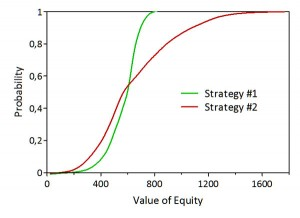In this case the distributions cross and there is no first order stochastic dominance: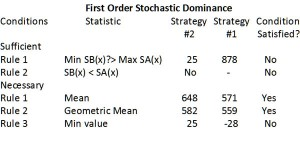To be able to determine the dominant strategy we have to make further assumptions on the utility function – U” ≤ (risk aversion) etc.

### N-th Order Stochastic Dominance.

With n-th order stochastic dominance we are able to rank a large class of strategies. N-th order dominance is defined by the n-th order distribution function: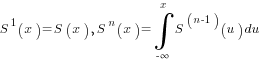where S(x) is the strategy’s distribution function.

Then strategy A dominates strategy B in the sense of n-order stochastic dominance – ADnB  – if:

SnA(x) ≤ SnB(x) , with at least one strict inequality and

EAU(X) ≥ EBU(X) , with at least one strict inequality,

for all U satisfying (-1)k U (k) ≤0 for k= 1,2,…,n. , with at least one strict inequality

The last assumption implies that U has positive odd derivatives and negative even derivatives:

U’  ≥0 → increasing wealth preference

U”  ≤0 → risk aversion

U’’’ ≥0 → ruin aversion (skewness preference)

For higher derivatives the economic interpretation is more difficult.

Calculating the n-th order distribution function when you only have observations of the first order distribution from Monte Carlo simulation can be difficult. We will instead use the lower partial moments (LPM) since (Ingersoll, 1987):

SnA(x) ≡ LPMAn-1/(n-1)!

Thus strategy A dominates strategy B in the sense of n-order stochastic dominance – ADnB  – if:

LPMAn-1 ≤ LPMBn-1

Now we have the necessary tools for selecting the dominant strategy of strategy #1 and #2. To se if we have 2nd order dominance, we calculate the first order lower partial moments – as shown in the graph below.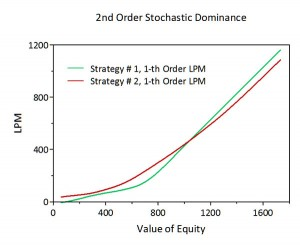Since the curves of the lower moments still crosses both strategies is efficient i.e. none of them dominates the other. We therefore have to look further using the 2nd order LPM’s to investigate the possibility of 3rd order dominance: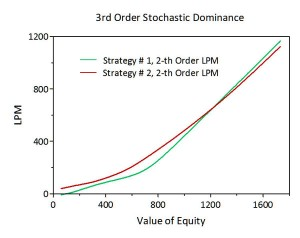However, it is first when we calculate the 4th order LPM’s that we can conclude with 5th order stochastic dominance of strategy #1 over strategy #2:We then have S1D5S2 and we need not look further since (Yamai, Yoshiba, 2002) have shown that:

If: S1DnS2 then S1Dn+1S2

So we end up with strategy #1 as the preferred strategy for a risk avers firm. It is characterized by a lower coefficient of variation (0.19) than strategy #2 (0.45), higher minimum value (160) than strategy#2 (25), higher median value (600) than strategy #2 (561). But it was not only these facts that gave us strategy #1 as stochastic dominant, because it has negative skewness (-0.73) against positive skewness (0.80) for strategy #2 and a lower expected value (571) than strategy #2 (648), but the ‘sum’ of all these characteristics.

### A digression

It is tempting to assume that since strategy #1 is stochastic dominant strategy #2 for risk avers firms (with U”< 0) strategy #2 must be stochastic dominant for risk seeking firms (with U” >0) but this is necessarily not the case.

However even if strategy #2 has a larger upside than strategy #1, it can be seen from the graphs of the two strategies upside potential ratio (Sortino, 1999):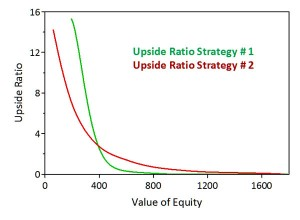that if we believe that the outcome will be below a minimal acceptable return (MAR) of 400 then strategy #1 has a higher minimum value and upside potential than #2 and vice versa above 400.

Rational firm’s should be risk averse below the benchmark MAR, and risk neutral above the MAR, i.e., they should have an aversion to outcomes that fall below the MAR . On the other hand the higher the outcomes are above the MAR the more they should like them (Fishburn, 1977). I.e. firm’s seek upside potential with downside protection.

We will return later in this serie to  how the firm’s risk and opportunities can be calculated given the selected strategy.

### References

Fishburn, P.C. (1977). Mean-Risk analysis with Risk Associated with Below Target Returns. American Economic Review, 67(2), 121-126.

Ingersoll, J. E., Jr. (1987). Theory of Financial Decision Making. Rowman & Littlefield Publishers.

Levy, H., (2006). Stochastic Dominance. Berlin: Springer.

Neumann, J., & Morgenstern, O. (1953). Theory of Games and Economic Behavior. Princeton: Princeton University Press.

Sortino, F , Robert van der Meer, Auke Plantinga (1999).The Dutch Triangle. The Journal of Portfolio Management, 26(1)

Yamai, Y., Toshinao Yoshiba (2002).Comparative Analysis of Expected Shortfall and Value-at-Risk (2): Expected Utility Maximization and Tail Risk. Monetary and Economic Studies, April, 95-115.

Series Navigation<< Valuation as a strategic tool